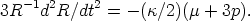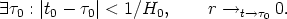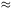Annu. Rev. Astron. Astrophys. 1984. 22: 157-184 Copyright © 1984 by Annual Reviews. All rights reserved

2.3. The Standard Models

The FLRW universe models (109, 45) are isotropic and spatially homogeneous, so their shear, vorticity, and acceleration are all zero. Thus the Raychaudhuri equation (8) reduces to(10)

By energy condition Equation 9b, this implies that the curve R(t) of the radius function against time always bends down. As the Universe is expanding at the present time t0, a singular origin must have occurred less than a Hubble time previously:(11a)

Because of the definition of R(t),

(A) at this time t0, the distance between all fundamental particles goes to zero like R(t).

At early times, Equations 4b and 5 show that T = T0(R0/R), where (from present observations of the CMBR) T03 K; thus,(11b)

This is the basic origin of the hot big-bang prediction in the FLRW universes:

(B) The Universe originates, and all physics breaks down, at the time t+0 where the temperature, energy density of matter, and space-time curvature diverge.

(For a more careful discussion, see 18.) Note that it is not merely the matter in the Universe that originates here: The space-time itself (and indeed the laws of physics) do not exist before this time. Now, Equations 4b, 5, and 10 show thatconverges; this implies that

(C) for each fundamental observer O, there exist particle horizons limiting possible communication with other fundamental observers in each direction at each time t; those further than the particle horizon will be unobservable by any radiation, and no causal influence from them can affect events in O's history up to the time t (45, 75, 85, 102).

The major properties characterizing the SHBB are (A)-(C) above and Equation 11, which follow from Equations 4, 5, 9, and 10. The standard results on the CMBR and element formation (i.e. the CMBR is relic radiation from the hot early phase when T > 103 K, and the light elements were synthesized when T109 K in the early Universe) can then be deduced from these properties (107, 109). If current ideas on the GUT are correct, at earlier times baryon creation will take place (42, 73, 116).

These models explain many features of the observed Universe in a satisfactory way, in particular the CMBR and primordial element abundances. However, they leave various other issues obscure, e.g. why the Universe is so nearly uniform, and yet not uniform [physical processes taking place after the creation of the Universe cannot be responsible because of the particle horizons (C); (38, 57, 64)], and why the density of matter is so close to the critical value separating recollapsing universes from ever-expanding universes (20, 38). Thus, one has the problem of explaining why the Universe started off from very special initial conditions. Additionally, the very concept of the creation of the Universe at such a singular beginning is philosophically objectionable to some scientists; they wish to find an alternative picture of its origin (23, 41, 102).